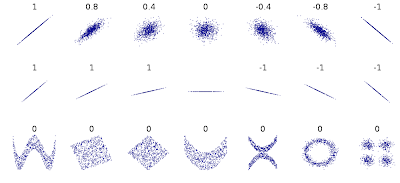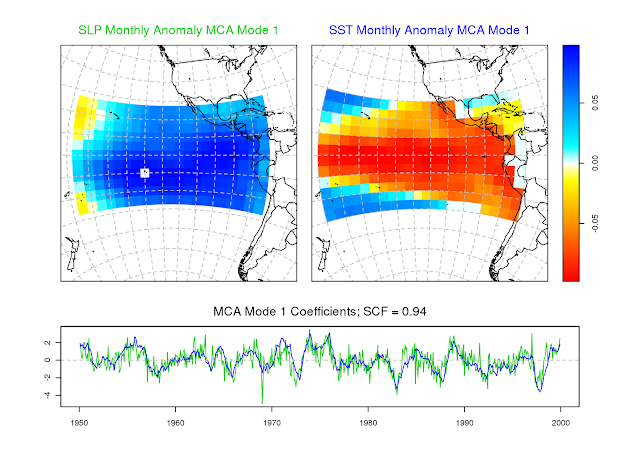## Monday, December 19, 2011

### Maximal Information Coefficient (MIC)Pearson r correlation coefficients for various distributions of paired data (Credit: Denis Boigelot, Wikimedia Commons)

A paper published this week in Science outlines a new statistic called the maximal information coefficient (MIC), which is able to equally describe the correlation between paired variables regardless of linear or nonlinear relationship. In other words, as Pearson's r gives a measure of the noise surrounding a linear regression, MIC should give similar scores to equally noisy relationships regardless of type.

## Tuesday, December 13, 2011

### Maximum Covariance Analysis (MCA)Maximum Covariance Analysis (MCA) (Mode 1; scaled) of Sea Level Pressure (SLP) and Sea Surface Temperature (SST) monthly anomalies for the region between -180 °W to -70 °W and +30 °N to -30 °S.  MCA coefficients (scaled) are below. The mode represents 94% of the squared covariance fraction (SCF).

Maximum Correlation Analysis (MCA) is similar to Empirical Orthogonal Function Analysis (EOF) in that they both deal with the decomposition of a covariance matrix. In EOF, this is a covariance matrix based on a single spatio-temporal field, while MCA is based on the decomposition of a "cross-covariance" matrix derived from two fields.